Goal Seek in Microsoft Excel 2010

In this article we will learn about how and where we can use to  Goal seek  to forecast the business on the basis of  present scenario in Microsoft Excel 2010.

If we know the result which we are looking for then we can find out the value of one cell by changing the value in another cell. In other words, Goal Seek tells the user to enter the input as to get a certain output.

Excel’s Goal Seek feature allows the user to change the data used in the formula in order to find out what the output will be.
Let us understand how PMT Function works:

The PMT function returns the payment amount of a loan based on an interest rate and a constant payment schedule.

PARAMETERS OF PMT FUNCTION

• interest_rate is the interest rate for the loan.
• number_payments is the number of payments for the loan.
• PV is the present value or principal of the loan.
• FV is optional. It is the future value or the loan amount outstanding after all payments have been made. If this parameter is omitted, the PMT function assumes a FV value of 0.

Type is optional. It indicates when the payments are due.

Type 0(Default): Payments are due at the end of the period.

Type 1: Payments are due at the beginning of the period.
Let us understand with an example:

We are calculating Loan Repayment Schedule. We have Interest Rate as 7%, Number of Payments as 60 (5 x 12), Loan Amount is \$ 60,000. Payment function calculates the payment for a loan based on constant payments with a constant interest rate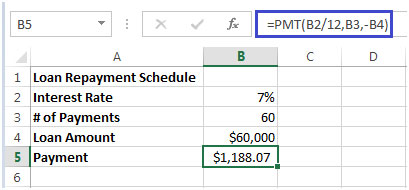Initial Payment every month is \$ 1,188.07. Let’s say we have less amount to be paid every month which is \$ 1,000

To calculate how many payments will get increased from 60, we will use Goal Seek function in Microsoft Excel.

• Click on Data Ribbon
• In Data Tools group, click on What-If Analysis
• Click on Goal Seek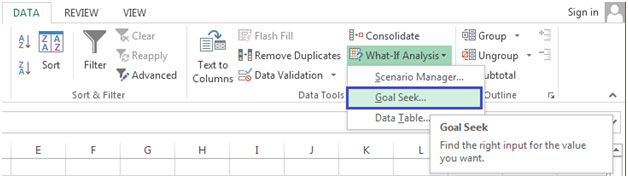• In Set cell, enter cell B5 where PMT function is calculating the monthly payment
• In To value, enter the amount that we want to pay
• In By changing cell field, enter cell B3 that is number of payments which will get increased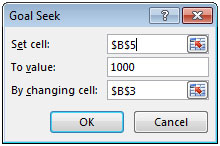• Click on OK button.
• We will get the following dialog box appear, click OK.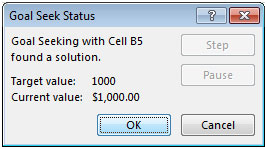• We will get below mentioned result as 74 approx (14=74-60).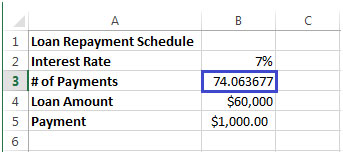• We have to pay 14 extra installments so that we can give only \$ 1,000 from our pocket instead of \$ 1,188.07
Terms and Conditions of use

The applications/code on this site are distributed as is and without warranties or liability. In no event shall the owner of the copyrights, or the authors of the applications/code be liable for any loss of profit, any problems or any damage resulting from the use or evaluation of the applications/code.